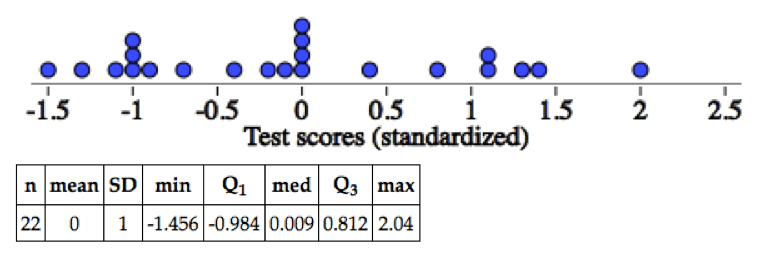Search

# Why Do We Standardize Normal Distributions?

Updated: Mar 6, 2018

What happens when a distribution is standardized? Let’s take a look at an example. Here is a distribution of test scores and the associated summary statistics:Now let’s see what happens to the distribution when we standardize all of the test scores by calculating z-scores for each test score:## Here is what we notice:

• The shape of the distribution stays the same. This means that the number of dots to the left of 70 (8 dots) is the same as the number of dots to the left of the standardized value for 70 = -0.51 (8 dots).

• We end up with a mean of 0.

• We end up with a standard deviation of 1.

The amazing fact is that these 3 noticings will be true for any distribution of values, and this includes normal distributions. So any normal distribution, with any mean and any standard deviation, can be standardized to a normal distribution with a mean of 0 and a standard deviation of 1 (the standard normal distribution).

## The connection to Table A

Let’s say we wanted to find the area to the left of 400 for a normal distribution with mean = 500 and SD = 60. Do we have a table of areas for the a normal distribution with mean = 500 and SD = 60? No…but someone could make one.

Or we wanted to find the area to the left of 14 for a normal distribution with mean = 30 and SD = 8. Do we have a table of areas for a normal distribution with mean = 30 and SD = 8? No…but someone could make one.

Someone is going to be very busy because they are going to have to make a table of areas for every possible normal distribution (infinite).

Solution: Take all of these infinite possibilities of normal distributions and standardize them all. Then make one table of areas for the standard normal curve. Voila. Table A! We just have to be convinced that the area given in Table A is the same as the area from the unstandardized distribution. But this is guaranteed by the fact that the unstandardized and standardized distributions have exactly the same shape (see the first noticing above).

So why do we standardize all of our normal distributions? So that we only have to have one area table, rather than an infinite number of area tables.

Of course, technology can find area under any normal curve and so tables of values are a bit archaic.  But I think that we should still bother with z-scores and Table A(and interpret z-scores like it’s your job).